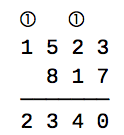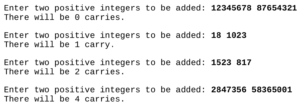## Carry Counts

Hello all!

It’s been a while since I’ve been able to post, but I wanted to cover another Bloomsburg problem from 2018 for my programming club.  The problem description is this:

When calculating the sum of two numbers by hand, we first add the digits in the 1’s position, and if the result overflows (i.e., is greater than 9) then we carry the leftmost 1 in the result to the 10’s position. This process is repeated at the 10’s position, then at the 100’s position, and so on. For example, if we are calculating 1523 + 817 by hand, we write:In this case, there are two carries (shown in circles).

Write a program that prompts the user for two positive integers and outputs the number of carries that would occur when performing addition by hand.

The following test cases illustrate the required I/O format.We’ll begin this problem by defining our variables and taking in some input.  Our input will be the two integers we will be adding, and then we will be putting them into a list.

carries = 0
int1 = int(raw_input("Enter two positive integers to be added: "))
int2 = int(raw_input())
ints = [int1, int2]

Next up we need to define our algorithm we will be using.   For this problem we will be using a new function called zfill(). The zfill() function fills a string up with zeroes on the left side until a specific length is reached.  We’ll also be using the .max() method to find the maximum value of the list, and then run the len() method on it to get the max length.  Doing this we can fill up both integers until they are the same length, allowing me to then work backwords through them, adding each value from right to left and counting the carries for each value.

length = len(max(ints))
add2 = int2.zfill(length)

Then we’ll use a for loop to loop through the length of the integers, moving from right to left and adding each value.  If the summed value is greater than 9, then the value will have a carry.  We also need to create a variable called extra, which I will use as the actual carry, because in a case like 89+11 the carry from the ones value causes the tens value to also increase by one, so we must account for it.  Otherwise if the summed value is less than or equal to nine, we do nothing and set extra equal to zero.

for i in range(length):
extra = 1
carries += 1
extra = 0

After we’ve iterated through the integers, we just need to check how many carries there were and print our statements accordingly for grammatical correctness.

if carries == 1:
print("There will be 1 carry.")
elif carries <= 0:
print("There will be no carries.")
else:
print("There will be ", carries, " carries")

And now we have a working solution to Bloomsburg 2018 Problem 3, Carry Counts.  I hope this helps. 🙂

Thanks for reading and have a wonderful day!
~ Corbin

## Vowel Shifter

Hello all!

This week we have another practice problem for the Bloomsburg Competition coming up.  This problem is a vowel shifter and the description goes as follows:

Write a program that prompts the user for a sentence and modifies it by shifting each vowel like this:
• a→ e
• e→ i
• i→ o
• o→ u
• u→ a
In other words, each “a” in the original sentence becomes an “e”, each “e” in the original sentence becomes an “i”, and so on, and similarly for capital letters.

We’ll start this program off by creating two lists for each of our vowel sets. These will be called vowelsupper and vowelslower.

vowels = ["a", "e", "i", "o", "u", "a"]
vowelsupper = ["A", "E", "I", "O", "U", "A"]

Next we need to grab our input from the user using phrase = str(raw_input("Enter a sentence.\n")) (Sidenote: The \n at the end of the sentence is an escape operator that just starts a new line.).  Next we need to create a way to iterate through our users input to find and replace vowels with our new shifted vowels.  We do this using a for loop.  A for loop is just a loop that repeats a set number of times and often is used to create a changing variable for the program. Inside this loop we want to use a conditional statement to check if each letter in the phrase is a vowel, and if it is a vowel we want to check if it is upper or lower case. After doing this we will shift the vowel and add the new vowel to our shifted phrase. Then we just repeat this process until we have iterated through the entire original string.

for i in range(len(phrase)):
if phrase[i] in vowelslower or phrase[i] in vowelsupper:
if phrase[i].islower():
shift += vowelslower[vowelslower.index(phrase[i])+1]
else:
shift += vowelsupper[vowelsupper.index(phrase[i])+1]
else:
shift += phrase[i]

Now we have all the main components needed to create our program.  After combining them all together our final code will look like this:

vowelslower = ["a", "e", "i", "o", "u", "a"]
vowelsupper = ["A", "E", "I", "O", "U", "A"]
shift = ""
phrase = str(raw_input("Enter a sentence.\n"))
for i in range(len(phrase)):
if phrase[i] in vowelslower or phrase[i] in vowelsupper:
if phrase[i].islower():
shift += vowelslower[vowelslower.index(phrase[i])+1]
else:
shift += vowelsupper[vowelsupper.index(phrase[i])+1]
else:
shift += phrase[i]
print(shift)

And now we have a working solution for Problem #2!  This solution is posted on my GitHub as well.

Thanks for reading and have a wonderful day!
~ Corbin

## Okapi and Preparing for the Bloomsburg Competition

Hello all!

I’ve been on a bit of a ‘hiatus’ lately, I’ve been busy with life things and haven’t had a chance to work on any posts here.  But a quick update, I won first place at regionals for the Pennsylvania Junior Academy of Science so I’m going to states in May and I’ll be making a post on that project soon.  I’ve also been preparing the programming club at my school for an upcoming competition at Bloomsburg University where we will be competing.  Because of this we have been doing practice problems and so I will be posting and explaining my solutions to them here.

Our first practice problem is called Okapi.  The problem description goes as follows:

The game of Okapi is played by rolling three dice. A payout in dollars is determined by the rolled numbers according to the following rule:

• If the three numbers are the same, the player wins the sum of those three numbers.
• If only two of the numbers are the same, the player wins the sum of the two equal numbers.
• For three different numbers, the player wins nothing.

Write a program that prompts the user for three dice rolls and outputs the payout.

We need to begin this problem by taking user input using rolls = input("Enter dice rolls: ") which prompts the user for input and sets rolls equal to their input. Next we need to parse out their answer into three separate rolls, this is rather easy and just a matter of indexing the user input. In order to do this we just need to create variables for each roll and then set them to the correct index of rolls using the following code: roll_one, roll_two, roll_three = int(rolls), int(rolls), int(rolls). Now that we have our rolls assigned we just need to use a bunch of conditional statements to determine the output.  Our final code will look like this:

def okapi():
rolls = input("Enter dice rolls: ")
roll_one, roll_two, roll_three = int(rolls), int(rolls), int(rolls)
if roll_one == roll_two and roll_two == roll_three:
print("The payout is $", roll_one*3, ".") elif roll_one == roll_two: print("The payout is$", roll_one+roll_two, ".")
elif roll_two == roll_three:
print("The payout is $", roll_two+roll_three, ".") elif roll_one == roll_three: print("The payout is$", roll_one+roll_three, ".")
else:
print("The payout is \$0.")

And now we have a working solution to problem #1!  Another solution can be found on my GitHub, it’s the same premise but just less readable.  I’ll most likely be posting around weekly again soon.

Thanks for reading and have a wonderful day!
~ Corbin